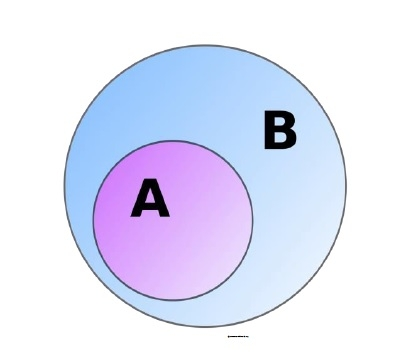# issubset() function in Python

In this article, we will learn the implementation and usage of issubset() function available in the Python Standard Library.

The issubset() method returns boolean True when all elements of a set are present in another set (passed as an argument) otherwise, it returns boolean False.

In the figure given below B is a subset of A. In case A & B are identical sets mean that A is a proper subset of B. This means both sets contain the same elements in them.## Syntax

<set 1>.issubset(<set 2>)

## Return value

boolean True/False

Now let’s look at an illustration to understand the concept.

## Example

Live Demo

A = {'t','u','t','o','r','i','a','l'}
B = {'t','u','t'}
C = {'p','o','i','n','t'}
print(B.issubset(A))
print(B.issubset(C))
A=set(str(A)+str(C))
print(C.issubset(A))

## Output

True
False
True

## Explanation

Here a check is made that all elements of B are contained in A which evaluates to be true. Similarly for the next statement output is produced.

Now we concatenated to sets to make it a subset forcefully by using typecasting as seen in the next statement.

Now let’s see what happens if we specify another type of iterable than set and pass it as an argument.

## Example

Live Demo

A = ['t','u','t','o','r','i','a','l']
B = {'t','u','t'}
C = ('p','o','i','n','t')
D = {'p','o','i','n','t'}
print(B.issubset(A))
print(B.issubset(C))
A=set(str(A)+str(C))
print(D.issubset(A))

## Output

True
False
True

## Explanation

Here we passed tuple, string and list iterables to the issubset() function. These types are implicitly converted to set type so as to get the desired output.

We must also observe that the argument outside the function must always of type <set> so that the interpreter must know that comparison is between two sets and not any other type.

## Conclusion

In this article, we learned how to use isubset() function in Python and what all types of arguments are allowed to be compared with the help of this function.